# The interactions of multiple particles in dense systems¶

## Introduction¶

Particle collisions are the dominating interaction happening in plasmas. There are two types of collisions:

1. Elastic collisions: collisions where the only exchange of energy involve kinetic energy; e.g. direct collisions, Coulomb collisions;
2. Inelastic collisions: collisions where the kinetic energy is lost to other precesses; e.g. ionization, recombination, excitation, polarizing collisions.

Collisions shoud always be understood in the framework of a projectile and a target. The projectile is aimed at a target in a certain way (this "aim" is called the impact parameter) and the target is define by it's surface area (also called cross-section).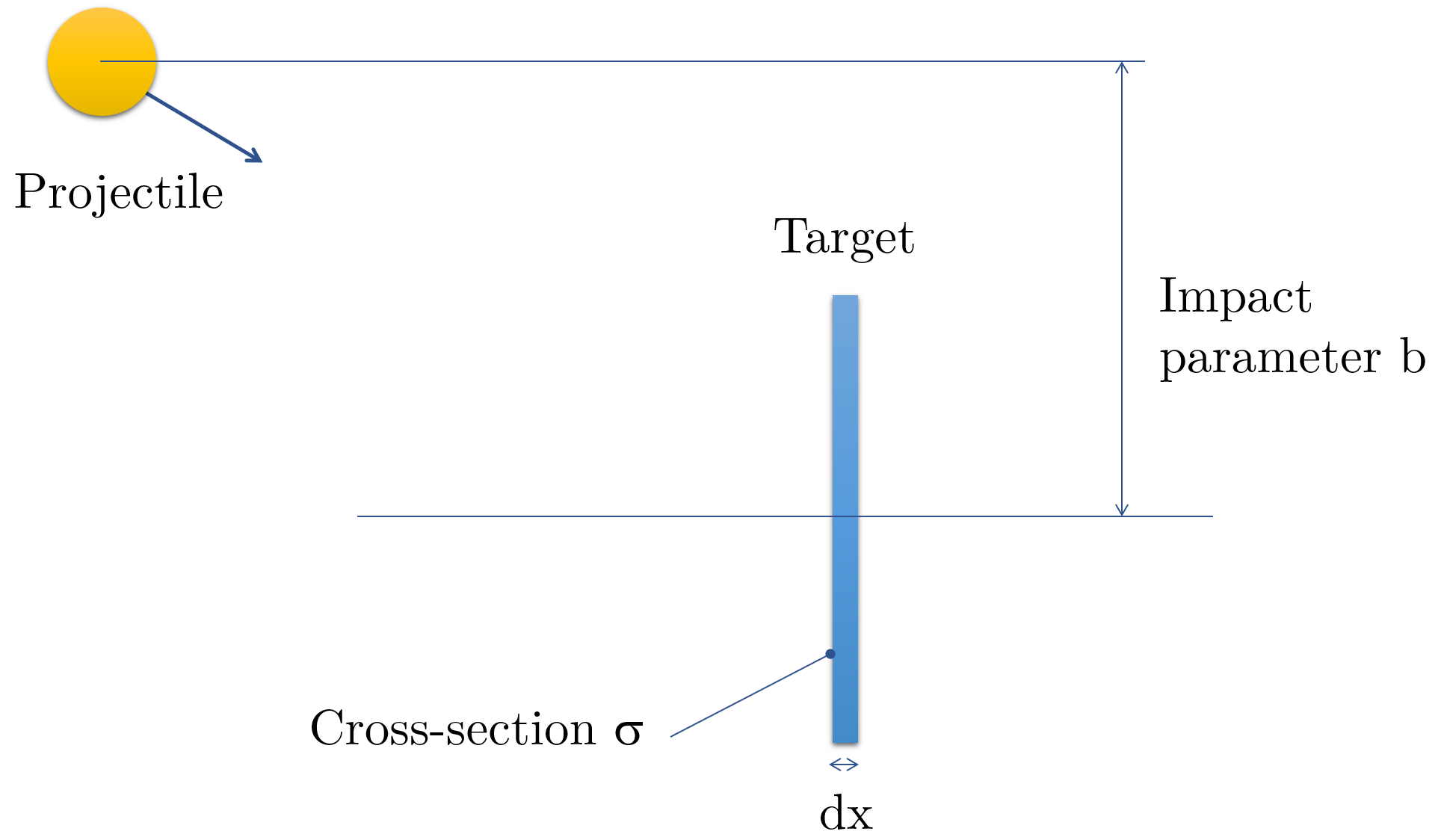The target can be one or many particles. The chance to hit the target is propertional to the cross-section ofthe target. A target with small cross-sectional area is hard to hit than a target with a larger cross-sectional area, keeping all other parameters constants.

Now, let's look at the simplest of all collisions, using the hard sphere model. We suppose both the projectile and its target are spheres. The projectile has a radius $r_p$ and the target has a radius $r_t$. Then collisions occur only when the impact parameter $b$ is $$b<r_p+r_t.$$ As seen by the projectile the target size, or cross-section is $$\sigma=\pi r^2.$$ While $\sigma$ is an area, it is not called $A$. This is due to the fact that for more complex models, like Coulomb collisions, it is much more complex that the actual area of the projectile-target system.

## Collision parameters¶

Let's start with $n$ projectiles incident on the target. The number of projectiles that have interacted with a target of thickness $dx$ is proportional to:

1. The density of the incident beam of projectiles: $n$
2. The density of particles inside the target: $n_g$
3. The thickness of the target: $dx$

The density $n$ is given in $m^{-3}$. For instance, a density $n=10^{10}m^{-3}$ means that there are $10^{10}$ particles per cubic meter. The constant of proportionality that link $dn$ to all the other parameters is called the cross-section (or cross-sectional area) of the interaction. It is given by $$dn=-\sigma n n_gdx\tag{3.1}$$ The minus sign takes into account that the incoming "beam" of projectiles is losing projectiles as the beam penetrates deeper into the target.

If we suppose that all the projectiles have the same velocity $\vec v$ then we can look at the particle flux $\vec \Gamma=n\vec v$ instead of the particle density $n$. We get a similar expression to Eq. $(3.1)$, namely $$d\vec\Gamma=-\sigma \vec \Gamma n_g d x$$

We can be slightly more general by supposing that the beam of projectile does have variations in the velocity and we use $<v>$ as the average beam speed, rather than the individual projectile speeds $v_i$. The most important parameters, besides the cross-section, are the collision frequency $\nu$ $$\nu=n_g\sigma<v>\tag{3.2}$$ $<v>$ here is the average speed of the ensemble of projectiles. The collision frequency corresponds to the number of times per second that a projectile hits a target. The other important quantity is the mean free path $\lambda$ $$\lambda=\frac{<v>}{\nu}=\frac{1}{n_g\sigma}\tag{3.3}$$ It is the average distance traveled by the projectiles between collisions. Finally, the mean time between interactions is given by $$\tau=\frac{\lambda}{<v>}\tag{3.4}.$$

Let's compute the mean free path in air, taking that the radius of each molecule is on the order of $R_{hs}=0.15nm$ (for $O_2$ or $N_2$).

At a pressure of 1 atmosphere, we can use $PV=NkT$ to compute the density of molecules. First, let's remember that $$n=\frac{N}{V}$$

In :
P=1e5 #in Pa
T=273 #in K
k=1.38e-23
n=P/(k*T)
R=0.15e-9 #in m
sigma=3.1416*(2*R)**2
mfp=1./(n*sigma)
print (mfp*1e9,'nm')


We will focus in the rest of the this chapter on elastic and inelastic Coulomb collisions, which often dominate plasmas. However the number of possible collision mechanisms in plasmas is quite rich:

1. hard sphere (yes they do happen)
2. polarizing collisions: when two molecules or atoms collide, their electrons can be rearrange and the molecules can acquire a dipole moment
3. charge exchange: a fast, positively charged ion encounters a slow neutral and "steals" its electron. Now the neutral is fast and the ion is slow, the charges have been exchange but with no significant loss in momentum.

## Collisions dynamics:¶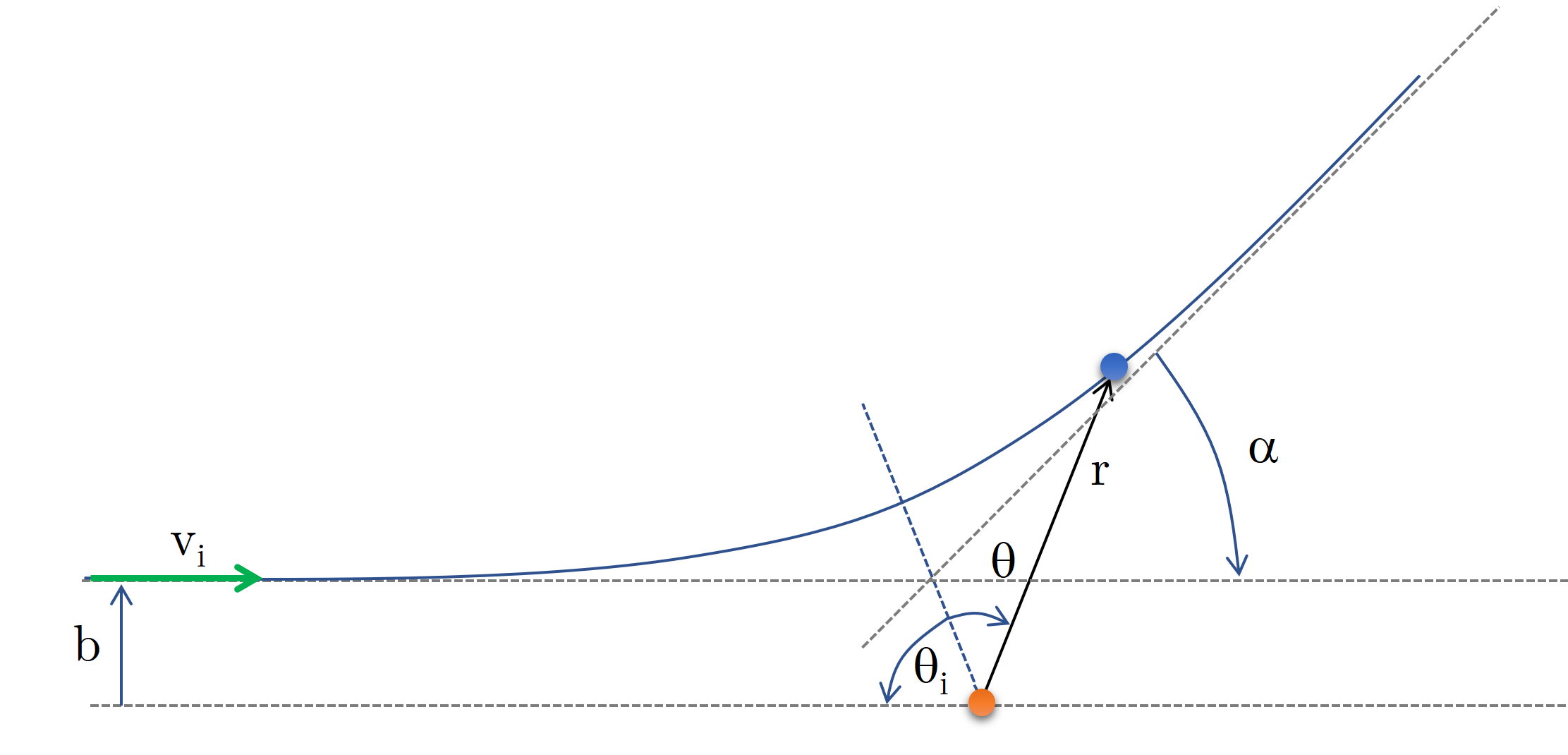Fig. 1: model of Coulomb collision with the orange mass not moving

Let's take two particles of mass $m_1$ and $m_2$ interacting via an action force $\vec F_{1->2}$ and a reaction force $\vec F_{2\rightarrow1}=-\vec F_{1\rightarrow2}$. If we suppose that the orange charge is not moving, the angles shown in the figure above can be used to describe the collision. Using the equation of motion for both particles we get $$m_1\ddot{\vec x}_1=\vec F_{2\rightarrow1}\\m_2\ddot{\vec x}_2=\vec F_{1\rightarrow2}$$ So we get $$\frac{m_1m_2}{m_1+m_2}(\ddot{\vec x}_1-\ddot{\vec x}_2)=\vec F_{2\rightarrow1}\tag{3.4}$$ We introduce here the reduced mass $m_R=\frac{m_1m_2}{m_1+m_2}$. If the force is a function of the distance $||\vec x_1-\vec x_2||$ (e.g. electrostatic force) then Eq. $(3.4)$ can be turned into $$m_R\ddot{\vec x}_R=\vec F_{2\rightarrow1}(\vec x_R)$$

This is the equation of motion of the center of gravity of the system $(1,2)$ with reduced mass $m_R$. Using the conservation of momentum $m_Rr\dot{\theta}=const.=m_Rbv_i$ and using $u=\frac{1}{r}$ and the fact that $\dot{\theta}=bv_ir^{-2}$ we get the the radial acceleration is $$\ddot{r}-r\dot{\theta}=-(bv_i)^2u^2\Big(\frac{d^2u}{d\theta^2}+u\Big)=\frac{F_{2\rightarrow1}}{m_R}$$ Using the fact that $$\vec F_{2\rightarrow1}=\frac{q_1q_2}{4\pi\varepsilon_0r^2}\hat{r}$$ we get $$\frac{d^2u}{d\theta^2}+u=-\frac{q_1q_2}{4\pi\varepsilon_0r^2}\frac{1}{m_Rb^2v_i^2}$$

which solution is simply $$u=A\cos(\theta)-\frac{q_1q_2}{4\pi\varepsilon_0r^2}\frac{1}{m_rb^2v_i^2}$$

Initially, $r\rightarrow-\infty$ then $u_i\rightarrow0$ so that $$A\cos(\theta)=\frac{q_1q_2}{4\pi\varepsilon_0r^2}\frac{1}{m_rb^2v_i^2}.$$ Of course, $\dot{ x}_i=-v_i=-bv_i\frac{du}{d\theta}|_{u=u_i}$. We can infer from this that $$\tan \theta_i=\frac{\sin \theta_i}{\cos\theta_i}=-\frac{b}{b_{90}}$$ using $b_{90}=\frac{q_1q_2}{4\pi\varepsilon_0r^2}\frac{1}{m_rv_i^2}$. When $b=b_{90}$ we have that $\tan \theta=-1$. For $\theta=-45^\circ$ we get $\alpha=90^\circ$.

Of course, we have written a function for this type of collisions in Ch. 1, called ODE_integration_E_with_charge. However, we can be in a situation where both projectile and target start to move. In this case we have to use the center of mass coordinates.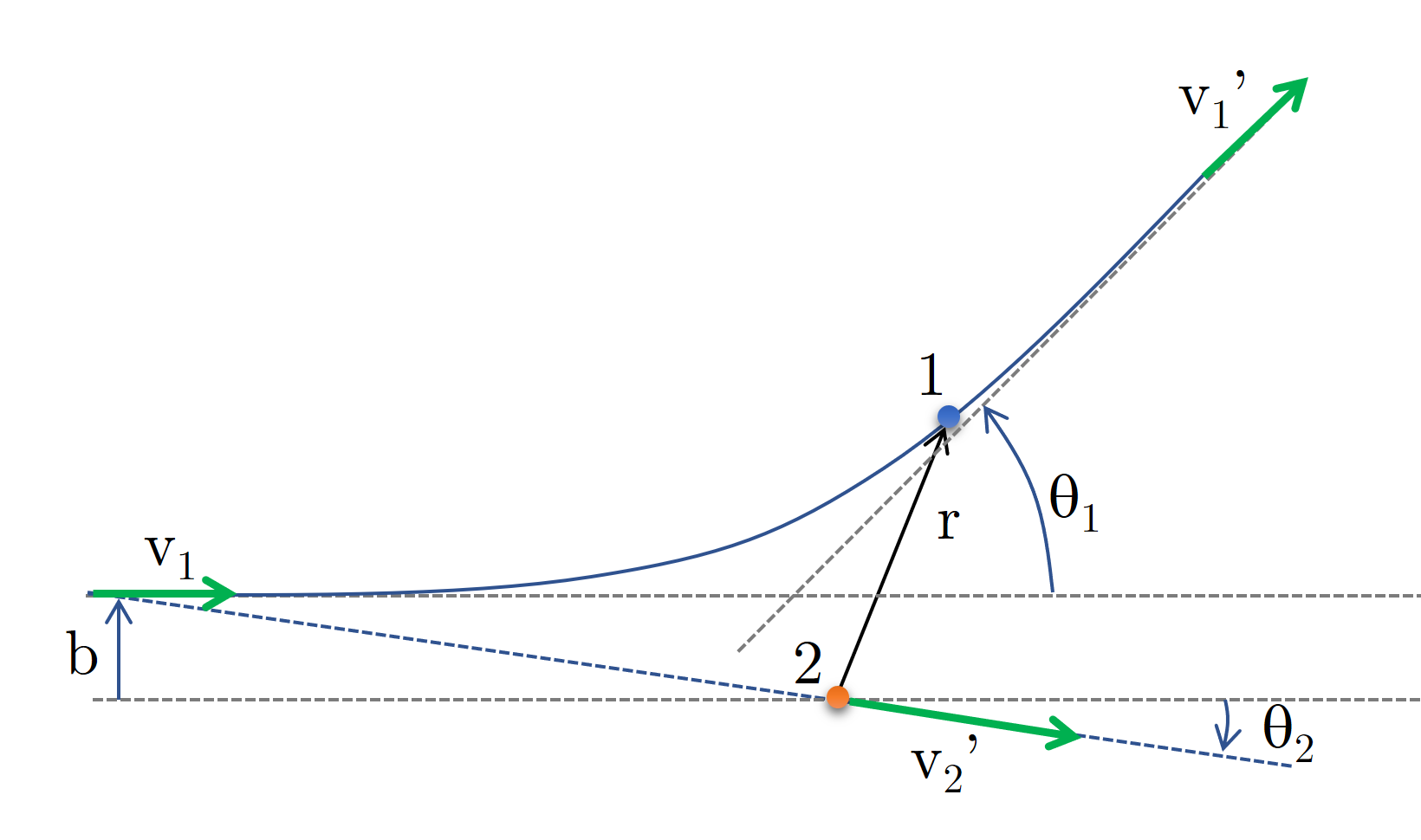Fig. 2: model of Coulomb collisions with both masses now free to move

We start with two particles of mass $m_1$ and $m_2$, with initial velocities $v_1$ and $v_2=0$ and impact parameter $b$. It is possible to use the model shown in Fig. 1 to describe the interaction. As before, the interaction here is only function of the distance between the two masses so we can use $\vec r=\vec x_1-\vec x_2$.

We now use the center of mass $$\vec R=\frac{m_1\vec x_1+m_2\vec x_2}{m_1+m_2}$$

With this center of mass comes a center of mass velocity $$\vec V=\dot{\vec r}=\frac{m_1\vec v_1+m_2\vec v_2}{m_1+m_2}.$$ Using the relative speed $v_R=\vec v_1-\vec v_2$ we get as before that $$m_R\dot{\vec v}_R=\vec F_{2\rightarrow1}$$

From momentum conservation we get that $$\tan\theta_1=\frac{\sin\alpha}{\frac{m_1}{m_2}\frac{v_R}{v_R'}+\cos(\alpha)}$$ and $$\tan\theta_2=\frac{\sin\alpha}{\frac{v_R}{v_R'}-\cos(\alpha)}$$ Because kinetic energy is conserved we get that $\vec v_R=\vec v_R'$. So the angle $\theta_1$ is given by $$\tan\theta_1=\frac{\sin\alpha}{\frac{m_1}{m_2}+\cos(\alpha)}$$ and $\theta_2$ by $$\theta_2=\frac{1}{2}(\pi-\alpha)$$ Let's compute the trajectory of both particles now.

Let's import our modules

In :
import math
from math import sqrt as sqrt
import numpy as np
import matplotlib.pyplot as plt
%matplotlib inline


And let's create a container that has all the information required to define a charged particle.

In :
class charged_particle:
m=9.10938356e-31 #mass
q=-1.60217662e-19 #charge
x=np.zeros(3) #location
v=np.zeros(3) #speed

In :
def binary_Coulomb_collision(charged_particle_0,charged_particle_1,time_frame,n_steps):
loc=np.zeros((3,n_steps,2))
loc[:,0,0]=charged_particle_0.x
loc[:,0,1]=charged_particle_1.x
v_old=np.zeros((3,2))
v_old[:,0]=charged_particle_0.v
v_old[:,1]=charged_particle_1.v
v_new=np.zeros((3,2))
dt=(time_frame-time_frame)/n_steps
for i in range(1,n_steps,1):
R=loc[:,0,0]-loc[:,0,1]
r=np.linalg.norm(R)
F=1/(4*np.pi*8.85e-12)*charged_particle_0.q*charged_particle_1.q/r**2*R/r
v_new[:,0]=F/charged_particle_0.m*dt+v_old[:,0]
v_new[:,1]=-F/charged_particle_1.m*dt+v_old[:,1]
loc[:,i,:]=v_new*dt+loc[:,i-1,:] #new position
v_old=v_new
return loc

In :
def plot_2D(r,n_interpolated=1,plot_size=3,plot_axes='axes'):
n = len(r[0,:,0])
k = n_interpolated
x_interpolated_0 = np.interp(np.arange(n * k), np.arange(n) * k, r[0,:,0])
y_interpolated_0 = np.interp(np.arange(n * k), np.arange(n) * k, r[1,:,0])
x_interpolated_1 = np.interp(np.arange(n * k), np.arange(n) * k, r[0,:,1])
y_interpolated_1 = np.interp(np.arange(n * k), np.arange(n) * k, r[1,:,1])
fig, ax = plt.subplots(1, 1, figsize=(plot_size, plot_size))
# Now, we draw our points with a gradient of colors.
ax.scatter(x_interpolated_0, y_interpolated_0, c=range(n*k), linewidths=0,
marker='o', s=2*plot_size, cmap=plt.cm.jet,)
ax.scatter(x_interpolated_1, y_interpolated_1, c=range(n*k), linewidths=0,
marker='o', s=2*plot_size, cmap=plt.cm.viridis,)
ax.axis('equal')
if (plot_axes!="axes"):
ax.set_axis_off()
else:
plt.xlabel("x (m)")
plt.ylabel("y (m)")

In :
cp1=charged_particle()
cp1.x=np.array([-9e-3,1e-3,0])
cp1.v=np.array([2,1,0])
cp2=charged_particle()
cp2.q=-1.6e-19
cp2.m=9e-31
time_frame=[0,1e-4]
n_steps=1000
r=binary_Coulomb_collision(cp1,cp2,time_frame,n_steps)
plot_2D(r,plot_size=8)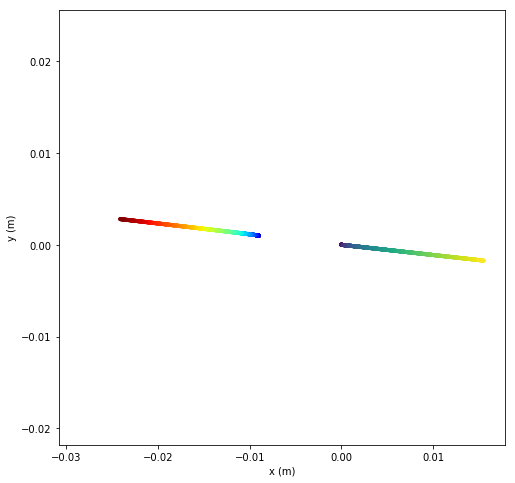## Plasma frequency and the Debye length¶

Because collisions are mostly deflections, with minimal exchange of energy, electrons are relatively free to move in between ions. In most cases, electrons are projectiles since the ions are much more massive and barely move on the electron timescale.

As a result, we can look at the ideal case where electrons have small oscillations near an equilibrium position. We can assume no collisions as long as the amplitude of motion is small.

### Plasma frequency¶

We start with a perfectly neutral plasma with ion density $n_0$. We displace the electrons by a small amount. The plasma is now electrically charged and an electric field develops such that $\vec \nabla . \vec E=\varepsilon_0 (n_0-n_e)$. We use $n_1=n_0-n_e<<n_0$. Using Newton's second law we get $$m_e\frac{dv_e}{dt}=-e\vec E.$$ Let's assume that we only have changes of density along the x-direction then we have $$\frac{\partial n_e}{\partial t}+\frac{\partial n_ev_e}{\partial x}=0$$ These equations formed a coupled system of partial differential equations. We can simplify the problem and assume that the density is oscillatory $n_e=\exp (ikx-i\omega t)$.

We now get $$ik\varepsilon_0E=-en_1\\-i\omega m_e v_e=-eE\\-i\omega n_1=-ikn_0v_e$$ What's the value of $\omega$?

We get $$\omega_e=\sqrt{\frac{n_0e^2}{\varepsilon_0m_e}}$$ The electron oscillates at a frequency $\omega_e$. This is called the plasma frequency. Let's compute this frequency is solid aluminum with density $n_0=6\times 10^{28}m^{-3}$.

In :
n_0=6e28
m_e=9.1e-31
epsilon_0=8.85e-12
e=1.6e-19
omega_e=(n_0*e**2/(epsilon_0*m_e))**0.5
print (omega_e)

1.3810305199653646e+16


Let's see the photon wavelength it corresponds to. We know that $\lambda=\frac{c}{\omega_e}$

In :
lambda_light=3e8/omega_e
print(lambda_light*1e9,'nm')

21.722908774494268 nm


This this in the UV range. This shows that aluminum (and metal in general) are good reflectors of UV light.

In :
def plasma_oscillations(n_0,th,particles,time_frame,n_steps):
loc=np.zeros((3,n_steps,len(particles)))
v_old=np.zeros((3,len(particles)))
for i in range(len(particles)):
loc[:,0,i]=particles[i].x
v_old[:,i]=particles[i].v
v_new=np.zeros((3,len(particles)))
dt=(time_frame-time_frame)/n_steps
for i in range(1,n_steps,1):
for j in range(len(particles)):
E=np.array([1/(4*np.pi*8.85e-12)*n_0*th*1.6e-19,0,0]) #electric field creating by a plane of positive charges
if (loc[0,i-1,j]<0):
E*=-1
F=particles[j].q*E
v_new[:,j]=F/particles[j].m*dt+v_old[:,j]
loc[:,i,j]=v_new[:,j]*dt+loc[:,i-1,j] #new position
v_old=v_new
return loc

In :
def plot_2D(r,n_interpolated=1,plot_size=3,plot_axes='axes'):
n = len(r[0,:,0])
k = n_interpolated
fig, ax = plt.subplots(1, 1, figsize=(plot_size, plot_size))
# Now, we draw our points with a gradient of colors.
for i in range(len(r[0,0,:])):
x_interpolated = np.interp(np.arange(n * k), np.arange(n) * k, r[0,:,i])
y_interpolated = np.interp(np.arange(n * k), np.arange(n) * k, r[1,:,i])
ax.scatter(x_interpolated, y_interpolated, c=range(n*k), linewidths=0,
marker='o', s=2*plot_size, cmap=plt.cm.jet,)
#compute autoscale parameters
xc=(r[0,:,:].max()+r[0,:,:].min())/2.
x_low=xc-(r[0,:,:].max()-r[0,:,:].min())/2.*1.1
x_high=xc+(r[0,:,:].max()-r[0,:,:].min())/2.*1.1
yc=(r[1,:,:].max()+r[1,:,:].min())/2.
y_low=yc-(r[1,:,:].max()-r[1,:,:].min())/2.*1.1
y_high=yc+(r[1,:,:].max()-r[1,:,:].min())/2.*1.1
#set autoscale parameters
ax.set_xlim(min(x_low,y_low),max(x_high,y_high))
ax.set_ylim(min(x_low,y_low),max(x_high,y_high))
#ax.axis('equal') #or this can be used
if (plot_axes!="axes"):
ax.set_axis_off()
else:
plt.xlabel("x (m)")
plt.ylabel("y (m)")

In :
N=30
cp=np.empty(N,dtype=object)
sp=np.linspace(-4e-3,4e-3,N)
for i in range (N):
cp[i]=charged_particle()
cp[i].x=np.array([-6e-3,sp[i],0])
cp[i].v=np.array([0,100000*(np.random.random_sample()-0.5),0])
time_frame=[0,50e-8]
n_steps=500
r=plasma_oscillations(1e19,1e-7,cp,time_frame,n_steps)
plot_2D(r,n_interpolated=20,plot_size=8)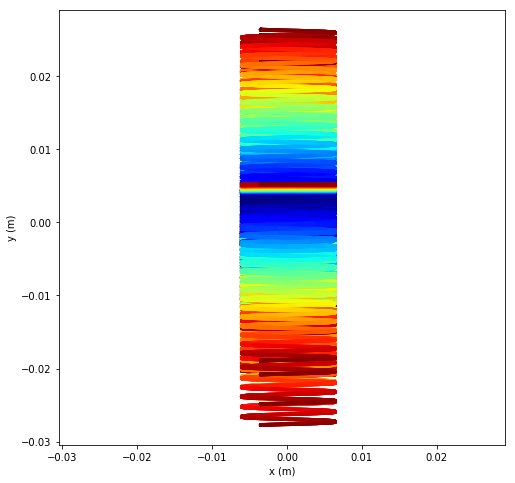### The Debye length¶

In general, plasmas are quasi neutral. They do not carry a net charge on large scales since they roughly have the same number of ions and electrons, i.e. $$Zn_i=n_e\tag{3.5}$$ where $Z$ is the ionization factor of ions (e.g. if 2 electrons have left an atom then the ionization factor of the resulting ion is $Z=2$). However, because of thermal motion, the plasma is not neutral locally. This means that there is a scale length $\lambda_D$ such that $Zn_i\neq n_e$. This length is the amplitude of the plasma oscillations just discussed but when electron motion is driven by thermal motion, i.e. $\lambda_D\omega_e=v_{T_{e}}$ or $$\lambda_D=\frac{v_{T_{e}}}{\omega_e}$$

## The cross-section for Coulomb collisions¶

In the previous section we saw how we connect the impact parameter and the speed and compute a deflection. We can then integrate for all deflections to get the total cross-section of Coulomb collisions. We can look at three types of collisions:

1. electron-electron $\sigma_{e-e}$
2. ion-ion $\sigma_{i-i}$
3. electron-ion $\sigma_{e-i}$

For each type of interaction, the cross-section will be different. Further, the deflections due to collisions can be strong or weak.

### Strong Coulomb collisions¶

Strong Coulomb collisions happens when the incident particles pass close to the target particles. In this case the deflection is large and the cross-section can be computed as follow. For strong collisions to happen we need to have the kinetic energy of the incident particle on the order of the Coulomb potential near the particle. If we look at electron(projectile)-ion(target) collisions $$\frac{Ze^2}{4\pi\varepsilon_0b}=\frac{m_ev_e^2}{2}$$ In this case, we can take the cross-section to be $\sigma=\pi b^2$ and we find that $$\sigma_{ei}=\pi\Big(\frac{Ze^2}{2\pi \varepsilon_0m_ev_e^2}\Big)^2$$ The collision frequency is found by using Eq. $(3.2)$ $$\nu_{ei}=n_i\sigma_{ei}v_e=\frac{Z^2e^4n_i}{4\pi\varepsilon_0^2m_e^2v_e^3}$$

Let's compute the cross-section $\sigma_{ei}$ and collision frequency $\nu_{ei}$ for electrons traveling at 10km/s and use a proton density of $n_i=10^{19}m^{-3}$.

In :
m_e=9.1e-31
Z=1
e=1.6e-19
epsilon_0=8.85e-12
v_e=10000
n_i=1e19
sigma=(Z*e**2/(2*np.pi*epsilon_0*m_e*v_e**2))**2*np.pi
print(sigma,'m**2')
print(sigma*n_i*v_e*1e-12,'THz')

8.040827412502484e-11 m**2
8.040827412502484 THz


### Small angle scattering Coulomb collisions¶

Rather than using the electron velocity, it is possible to use the electron temperature $T_e$. This is done by supposing that all particle have the same "temperature" so that $$\frac{1}{2}m_ev_e^2=eT_e$$ and for the ions $$\frac{1}{2}m_iv_i^2=eT_i$$

where $T_i$ and $T_e$ and in volt (not in Kelvin, not in Celsius). In plasmas, temperatures are really high, so it is usually given in volt, not in K. If you need to do a conversion, remember that $$k_BT(K)=eT(V).$$ $k_B$ is the Boltzmann constant (i.e. $1.38\times 10^{-23}$). We are using this weird unit for temperature because the temperature goes high quickly in plasmas. We would end up with very large number. Let's compute the temperature in K of a plasma inside the solar corona with temperature $T_e=100eV$

In :
T_e=100 ##in eV
k=1.38e-23
e=1.6e-19
T_K=e*T_e/k #in K
print(T_K,'K')

1159420.2898550723 K


Large angle deflections come from the accumulation of small angle scattering of many collisions, leading to an inflation of the Coulomb cross-section by $\ln \Lambda$, called the Coulomb logarithm, which is $$\ln \Lambda=\ln\Big(\frac{2\lambda_D}{b}\Big)$$ Typical values for $\ln \Lambda$ are between 10 and 15. So the collision frequency becomes $$\nu_{ei}=\frac{Z^2e^4n_i\ln\Lambda}{4\pi\varepsilon_0^2m_e^2v_e^3}$$ In this case we need to use statistical mechanics (we are using temperature after all). This brings forth the average collision rate $<\nu_{ei}>$ rather than $\nu_{ei}$ $$<\nu_{ei}>=\frac{\sqrt{2}n_iZ^2e^4\ln\Lambda}{12\pi^{3/2}\varepsilon_0^2m_e^{1/2}(eT_e)^{3/2}}$$ We can conversely compute the electron-electron frequency $\nu_{ee}$ after forming an average to use the electron temperature $$<\nu_{ee}>=\frac{n_ee^4\ln\Lambda}{\varepsilon_0^2m_e^{1/2}(eT_e)^{3/2}}$$ Note that the ratio between $<\nu_{ee}>$ and $<\nu_{ei}>$ is on the order of $n_iZ^2/n_e$. We can also apply the same method to compute $<\nu_{ii}>$, i.e. $$<\nu_{ii}>=\frac{n_iZ^4e^4\ln\Lambda}{12\pi^{3/2}\varepsilon_0^2m_i^{1/2}(eT_i)^{3/2}}$$ Note that the factor $\sqrt{2}$ is gone because we look at ions of the same mass. As a results the reduced mass is simply $m_R=\frac{m_i^2}{2m_i}=\frac{m_i}{2}$

Note: here all temperatures are in $V$

## Particle in cell¶

It is possible to approximate Coulomb collisions by grouping the particles together, in clumps, computing their charge distribution on a grid, advancing the electrostatic quantities on this grid using Maxwell's equations, and then computing the force caused by the "grid" electric field on each particle clump. This is the spirit of the so called Vlasov equation, that we will see i nthe next chapter. This method is know as particle-in-cell or PIC.

While this method uses both electric and magnetic fields, we focus on electric fields only here.

In :
import pyamg
import scipy

In :
class charged_particle:
m=9.10938356e-31 #mass
q=-1.60217662e-19 #charge
x=0. #location
y=0. #location
vx=0. #speed
vy=0. #speed

In :
class grid_2D:
Nx=10
Ny=10
x=np.zeros(Nx)
y=np.zeros(Ny)
data=np.zeros((Nx,Ny,2))

In :
def charge_deposition(charged_particles,grid,tiny):
charge_density=np.zeros((grid.Nx,grid.Ny))
dist=np.zeros((2,2))
val=np.zeros((2,2))
dx=grid.x-grid.x
dx=grid.y-grid.y
for n in range (len(charged_particles)):
ig=int((charged_particles[n].x-grid.x)/(grid.x[grid.Nx-1]-grid.x)*(grid.Nx-1))
jg=int((charged_particles[n].y-grid.y)/(grid.y[grid.Ny-1]-grid.y)*(grid.Ny-1))
if (ig>=grid.Nx-2):
ig=grid.N_x-2
if (jg>=grid.Ny-2):
jg=grid.Ny-2
for i in range (2):
for j in range (2):
dist[i,j]=sqrt((charged_particles[n].x-grid.x[ig+i])**2+(charged_particles[n].y-grid.y[jg+j])**2)
ave=0
for i in range (2):
for j in range (2):
val[i,j]=1./(dist[i,j]+tiny)
ave+=val[i,j]
for i in range (2):
for j in range (2):
charge_density[ig+i,jg+j]+=val[i,j]*charged_particles[n].q/ave
return charge_density/8.85e-12/abs((grid.x-grid.x)*(grid.y-grid.y))

In :
def comp_E(charge_density,grid):
Asize=len(charge_density[:,0])*len(charge_density[0,:])
b=np.zeros(Asize)
for i in range (len(charge_density[:,0])):
for j in range (len(charge_density[0,:])):
k=j+i*len(charge_density[0,:])
b[k]=charge_density[i,j]
stencil = [ [-1,-1,-1],[-1,8,-1],[-1,-1,-1] ]
A = pyamg.gallery.stencil_grid(stencil, (len(charge_density[:,0]),len(charge_density[0,:])), dtype=float, format='csr')
B = np.ones((A.shape,1))
ml = pyamg.smoothed_aggregation_solver(A,B,max_coarse=10)
#print (ml)
residuals=[]
x0 = scipy.rand(A.shape,1)
x = ml.solve(b=b,x0=x0,tol=1e-10,residuals=residuals,accel='cg')
#(residuals[-1]/residuals)**(1.0/len(residuals))
solution=np.zeros((len(charge_density[:,0]),len(charge_density[0,:])))
grid.data=np.zeros((len(charge_density[:,0]),len(charge_density[0,:]),2))
for i in range (len(charge_density[:,0])):
for j in range (len(charge_density[0,:])):
k=j+i*len(charge_density[0,:])
solution[i,j]=x[k]
for i in range (1,len(charge_density[:,0])-1):
for j in range (1,len(charge_density[0,:])-1):
grid.data[i,j,1]=-(solution[i+1,j]-solution[i-1,j])/2.
grid.data[i,j,0]=-(solution[i,j+1]-solution[i,j-1])/2.
return solution

In :
T_e=.1
n_e=1e19
m_e=9e-31
e=1.6e-19
v_e=sqrt(2*e*T_e/m_e)
omega_e=sqrt(n_e*e**2/(8.85e-12*m_e))
lambda_D=v_e/omega_e
length=8*lambda_D
dt=length/v_e/4
T=2*3.1416/omega_e
N=int(n_e**(1/3)*length)*10
time_frame=[0,25*T]
n_steps=int((time_frame-time_frame)/dt)
print('v_e=',v_e,'m/s')
print('lambda_D=',lambda_D,'m')
print('T=',T,'s')
print('number of particles in the plane:',N,'x',N)
print('time step',dt)
print('number of cycles',n_steps)

v_e= 188561.80831641264 m/s
lambda_D= 1.0517841983981314e-06 m
T= 3.5047237478152256e-11 s
number of particles in the plane: 180 x 180
time step 1.1155856085482638e-11
number of cycles 78

In :
box=np.array([-length,length,-length,length])*0.75
E_grid=grid_2D
E_grid.Nx=N
E_grid.Ny=N
E_grid.x=np.linspace(-length,length,N)
E_grid.y=np.linspace(-length*2,length,N)
E_grid.data=np.zeros((E_grid.Nx,E_grid.Ny,2))
rho_grid=grid_2D
rho_grid.Nx=N
rho_grid.Ny=N
rho_grid.x=np.linspace(-length,length,N)
rho_grid.y=np.linspace(-length,length,N)
rho_grid.data=np.zeros((rho_grid.Nx,rho_grid.Ny))
cp=np.empty(0,dtype=object)
l=0
Np=10000

for i in range (Np):
cp=np.append(cp,charged_particle())
cp[l].m=1.67e-27
cp[l].x=box+(box-box)*np.random.random_sample()
cp[l].y=box+(box-box)*np.random.random_sample()
cp[l].q*=-1
cp[l].vx=0
cp[l].vy=0
l+=1

for i in range (Np):
cp=np.append(cp,charged_particle())
rd=np.random.random_sample()
vx=v_e/sqrt(2)*math.cos(2*math.pi*rd)
vy=v_e/sqrt(2)*math.sin(2*math.pi*rd)
cp[l].x=box+(box-box)*np.random.random_sample()
cp[l].y=box+(box-box)*np.random.random_sample()
cp[l].vx=vx
cp[l].vy=vy
l+=1

In :
cd=charge_deposition(cp,E_grid,1e-5)
sol=comp_E(cd,E_grid)
fig, ax = plt.subplots(figsize=(10, 10))

im = plt.imshow(np.flip(np.flip(np.hypot(E_grid.data[:,:,0],E_grid.data[:,:,1]),0),1), cmap=plt.cm.jet, extent=box)
#im = plt.imshow(np.flip(np.flip(cd,0),1), cmap=plt.cm.jet, extent=box)
im.set_interpolation('bilinear')

cb = fig.colorbar(im)
plt.xlabel('x', rotation=0)
plt.ylabel('y', rotation=90)
cb.ax.set_ylabel('E', rotation=-90)
cb.ax.yaxis.set_label_coords(6, 0.5)
plt.show()

D:\Anaconda3\lib\site-packages\pyamg\gallery\stencil.py:114: FutureWarning: Using a non-tuple sequence for multidimensional indexing is deprecated; use arr[tuple(seq)] instead of arr[seq]. In the future this will be interpreted as an array index, arr[np.array(seq)], which will result either in an error or a different result.
diag[s] = 0
D:\Anaconda3\lib\site-packages\pyamg\gallery\stencil.py:110: FutureWarning: Using a non-tuple sequence for multidimensional indexing is deprecated; use arr[tuple(seq)] instead of arr[seq]. In the future this will be interpreted as an array index, arr[np.array(seq)], which will result either in an error or a different result.
diag[s] = 0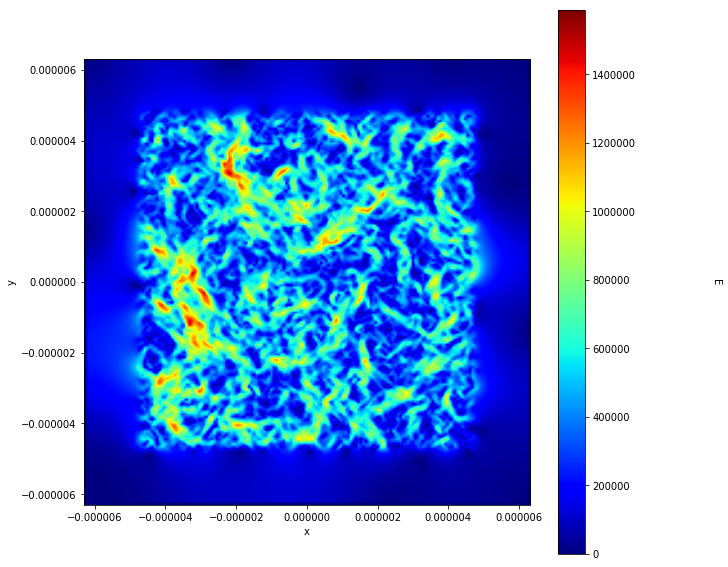## Problems:¶

### Problem 1¶

1. Write a version of binary_Coulomb_collisions that can handle any arbitrary number of particles.
2. Modify plot_2D function so that you can print the results.

### Problem 2¶

1. Write a function that shows the trajectory of many electrons inside the lattice of fixed ions and use the right boundary conditions to generate plasma oscillations
2. Measure the oscillation frequency and compare it to analytical results.

### Problem 3¶

Compute the collisions frequencies $\nu_{ee}$, $\nu_{ei}$ and $\nu_{ii}$ for the following hydrogen plasmas:

1. Earth ionosphere: $n_e=10^{11}m^{-3}$ and $T_e=10meV$
2. Solar corona plasma: $n_e=10^{15}m^{-3}$ and $T_e=100eV$
3. Solar wind plasma: $n_e=10^{7}m^{-3}$ and $T_e=10eV$
4. A fusion reactor: $n_e=10^{23}m^{-3}$ and $T_e=10keV$
5. A Z-pinch: $n_e=10^{25}m^{-3}$ and $T_e=500eV$
6. A laser plasma: $n_e=10^{28}m^{-3}$ and $T_e=500eV$

Note that we use hydrogen, so $n_i=n_e$ and $Z=1$. Where necessary we will suppose that $T_i=T_e$

### Problem 4¶

1. Compute the Debye length $\lambda_D$ for several densities between $10^{19}m^{-3}$ and $10^{20}m^{-3}$ and for several temperatures between $0.1eV$ and $1eV$.

Using a version of binary_Coulomb_collisions that can handle any arbitrary number of particles, generate a hydrogen plasma inside a 2-dimensional box, using the previous densities and temperatures, supposing that $n_i=n_e$ and that the ions do not move. Make sure that the box is much larger than the large Debye length compute in question 1. After several time steps:

1. Plot the average electric field inside the box for each density and temperature (Hint: sum the electric fields over time as vectors, then take the strength of this average electric field)
2. Compute the Debye length $\lambda_D$ using the information you got from this electric field
3. Compare it to the theoretical values computed in 1.

### Problem 5¶

Using the binary collision function defined in this chapter, plot the distribution function of 25 protons and 25 electrons locked inside a box of size 2mmx2mm, randomly distributed, for each of the following initial parameters:

1. $v_i=v_e=1000\,m/s$, all with random directions
2. $v_i=v_e/10=100\,m/s$, all with random directions

Note that we will suppose that there is no displacement along the Z-direction and that all particles reflect back inside the box when they hit the walls of the box.

### Problem 6¶

Compute numerically the total number of particles for an infinitely large box where the particles inside have the following distribution function: $$f(\vec x,\vec v,t)=A_0\exp\Big(-\frac{x^2+y^2+z^2}{L_0^2}\Big)\exp\Big(-\frac{v_x^2+v_y^2+v_z^2}{v_0^2}\Big)$$ where $A_0=200\,particles/m^6s^3$, $L_0=2 m$ and $v_0=0.1\,m/s$. Compare the numerical answer with the analytical answer using the fact that $$\int_{-\infty}^{+\infty}\exp(-x^2)dx=\sqrt{\pi}.$$

© Pierre Gourdain, The University of Rochester, 2020

In [ ]: